## Overview of questions:

1. Specify all forms of Fourier series, which you know
2. Specify equations for calculation of coefficients of sine-cosine and rectangular form Fourier series
3. Specify equations for calculation of coefficients of complex exponential form Fourier series
4. Define effective value of a periodic current (voltage) (root mean square value)
5. State effective value of a periodic current (voltage) that is represented by a Fourier series
6. Define active power of periodic non-sinusoidal voltage and current for general two-port
7. State formula for calculation of the active power of periodic non-sinusoidal voltage and current of two-port, if voltage and current are expressed by means of Fourier series coefficients
8. State formula for calculation of the reactive power of periodic non-sinusoidal voltage and current of two-port, if voltage and current are expressed by means of Fourier series coefficients
9. State formula for calculation of the apparent power of periodic non-sinusoidal voltage and current of two-port, if voltage and current are expressed by means of Fourier series coefficients
10. State formula for calculation of the deformation power of periodic non-sinusoidal voltage and current of two-port
11. Describe how to derive Fourier transform of non-periodic variable by means of generalization of formula for calculation of coefficients of the complex exponential form of the Fourier series of periodic variable
12. State for which classes of non-periodic signals their Fourier transform exist
13. Describe how is possible to extend class of functions, transformable by Fourier transform using Laplace transform
14. Write an Laplace transform of a function derivative
15. Write an Laplace transform of a function integral
16. Evaluate impedance of linear passive two-port passing through by sinusoidal currentwith voltage across it in sinusoidal steady state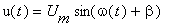17. Evaluate impedance of general linear passive two-port passing through by general time function current i(t) and with general time function voltage u(t) across it, if i(t) = 0 for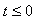and u(t) = 0 for18. Evaluate admittance of linear passive two-port passing through by sinusoidal current it.gif with voltage across it in sinusoidal steady state19. Evaluate admittance of general linear passive two-port passing through by general time function current i(t) and with general time function voltage u(t) across it, if i(t) = 0 forand u(t) = 0 for20. Evaluate voltage transfer function of linear passive circuit supplied by voltage source u1h.gif, if its sinusoidal steady state output voltage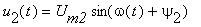21. Evaluate voltage transfer function of general linear passive circuit supplied by general time function voltage source u1(t) if its output voltage is u2(t). Assume, that u1(t) = 0 forand u2(t) = 0 for22. Draw the series equivalent circuit diagram of a charged capacitor, which contains operational impedance and equivalent source of initial condition.
23. Draw the parallel equivalent circuit diagram of a charged capacitor, which contains operational admittance and equivalent source of initial condition.
24. Draw the series equivalent circuit diagram of an inductor passing by initial current iL(0+), which contains operational impedance and equivalent source of initial condition.
25. Draw the parallel equivalent circuit diagram of an inductor passing by initial current iL(0+), which contains operational admittance and equivalent source of initial condition.
26. Write relations between Laplace transforms of current and voltage for a linear resistor.
27. Write relations between Laplace transforms of current and voltage for a charged capacitor.
28. Write relations between Laplace transforms of current and voltage for an inductor passing by initial current iL(0+).
29. Linear circuit is described by a diferential equation of the formWrite the general solution of this equation and its modification for excitation by constant (DC sources) or by sinusoidal function.
30. Linear circuit is described by a diferential equation of the formWrite the general solution of this equation and its modification for excitation by sinusoidal function.
31. Linear circuit is described by a diferential equation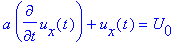Find its solution for general initial condition ux(0+).
32. Linear circuit is described by a diferential equation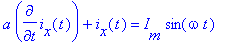Find its solution if ix(0) = 0.
33. Linear circuit is described by a diferential equation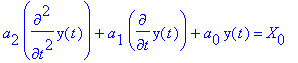Find its solution if a characteristic equation has two different real roots l1, 2 , X0 = const.
34. Linear circuit is described by a diferential equationFind its solution if a characteristic equation has a repeated root l.
35. Linear circuit is described by a diferential equationFind its solution if a characteristic equation has a complex conjugated roots l1 = -a + j w, l2 = -a + j w.
36. Linear circuit contains m capacitors, which can't be grouped together, and n inductors, which can't be grouped together. For this circuit describe difference between energetic initial conditions and mathematical initial conditions.
37. Write the physical meaning of unit step response of a linear circuit.
38. Evaluate generally unit step response of a linear circuit with input variable x1(t) and output variable x2(t).
39. Evaluate unit step response of a linear circuit with input variable x1(t) and output variable x2(t) and write, how is possible to measure it.
40. Evaluate generally unit impulse response of a linear circuit with input variable x1(t) and output variable x2(t).
41. Evaluate unit impulse response of a linear circuit with input variable x1(t) and output variable x2(t) and write, how is possible to measure it.
42. Which condition must met poles of a transfer function, if a unit impulse response should be finite function (stable circuit, poles are only on the left-hand side of the complex plane).
43. Write, which parts (function types) can contain unit impulse characteristic of a stable lumped circuit.
44. Write the relation between unit step characteristic and unit impulse characteristic of the same circuit.
45. For circuit with transfer function P(p) = U2(p)/U1(p) write general formulas for calculation of the modulus and phase response (evaluation of a variables on vertical axeses of that characteristics).
46. Given the circuit with the transfer function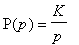. Draw the asymptotic magnitude and phase frequency response in logarithmic coordinates.
47. Given the circuit with the transfer function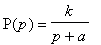. Draw the asymptotic magnitude and phase frequency response in logarithmic coordinates.
48. Given the circuit with the transfer function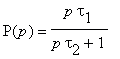. Draw the asymptotic magnitude and phase frequency response in logarithmic coordinates.
49. Given the circuit with the transfer function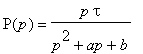. Draw the asymptotic magnitude and phase frequency response in logarithmic coordinates, if the transfer function has 2 real negative poles p1, p2.
50. Given the circuit with the transfer function. Draw the asymptotic magnitude and phase frequency response in logarithmic coordinates, if the transfer function has 2 real negative poles p1, p2.
51. Given the circuit with the transfer function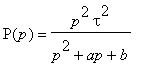. Draw the asymptotic magnitude and phase frequency response in logarithmic coordinates, if the transfer function has 2 real negative poles p1, p2.
52. Given the circuit with the unit step response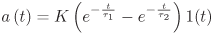. Draw the asymptotic magnitude and phase frequency response in logarithmic coordinates.
53. Given the circuit with the unit impulse response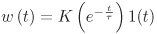. Draw the asymptotic magnitude and phase frequency response in logarithmic coordinates.
54. Given the circuit whose unit impulse response is w(t). Find in general its response u2(t) to the input voltage u1(t).
55. Given the circuit whose unit step response of its voltage transfer function is a(t). Find in general its response u2(t) to the input voltage u1(t) = Um 1(t) - Um 1(t-t0) (rectangular pulses of the width t0).
56. Draw the elementary section of a homogeneous transmission line and deduce its basic line equations.
57. Write the wave equation of a homogeneous transmission line and its solution.
58. Prove, that wave equations are satisfied by the arbitrary function u(x,t) = f1(x-vt) + f2(x+vt) for lossless transmission line.
59. Write the equations for caltulation of the wave resistance and the wave propagation speed along the line (wave velocity) of the homogeneous lossless transmission line if you know primary parameters per unit length (L and C).
60. Define the reflection coefficient at the point of connection of two transmission lines which have different wave resistances and write equation for its calculation.
61. Define the transmission coefficient at the point of connection of two transmission lines which have different wave resistances and write equation for its calculation.
62. Find the reflection coefficient at the end of the open-circuited and short-circuited transmission line.
63. Find the reflection coefficient at the end of the transmission line loaded by the resistor Rs with resistivity equal to the line's wave resistivity R0.